## Year 6 resource of the week: factors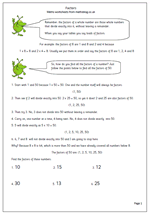Understanding factors and multiples is something many children fail to grasp. Here we have a page on factors and how to find all the factors of a number. The process is fairly straightforward, but can be quite time consuming. A good knowledge of tables and division is also needed. So if children’s knowledge of tables is weak, if they find division difficult and if they have little staying power then it is unlikely that they will enjoy trying to find factors of numbers!

One point that even brighter children take a while to understand is that you only need to continue to divide up to when the number squared is larger than the original number eg to find the factors of 62 you only need to divide 62 by numbers up to 7 because eight eights are 64. It is a good idea to spend some time explaining and showing the logic of this to children.

This can be found in our Year 6 Understanding Number section.

Factors 1

## Resource of the Week: Year 5 Number Challenge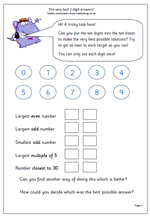I really like this challenge, partly because there is no, one right way to answer it and partly because it really makes children think.

There are 10 digits, from zero to nine to be placed in the 10 boxes in such a way that the targets can be matched as closely as possible. The catch is that each number can only be used once!

Now the obvious way to start is to make 98 the largest even number, but immediately that means that you can not have 97 as the largest odd number. By the time you reach the last target, number closest to 30, you have only two digits left and only two choices!

But what counts as the best possible answers? This is as big a challenge, if not bigger. I have had a class try to make a set of rules to try to be as fair as possible, but it involved a great deal of addition and subtraction. One group made a set of rules that went like this:

1. Find the difference between 98 and the answer given.

2. Find the difference between 99 and the answer given.

This will give your total so far – the larger the total, the worse you have done.

I won’t continue with this as it might spoil the fun!

This page can be found in our Year 5, Using and Understanding Maths category.

## Subtracting mentally in Year 4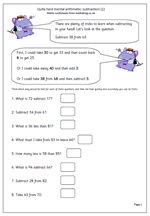Making the correct choice in deciding how to work out an answer is the key to fast mental arithmetic. Many children are unaware that there are often several ways of working out a calculation and that those who choose the best methods find maths easier and are able to answer questions more quickly and more accurately.

Let’s look at ‘Subtract 38 from 63.

This should be done, ‘in your head’ and will involve several stages, depending on the method used.

First, I could take 30 from 63 to leave 33. Then I could count back 8 from 33 which gives me 25.

Secondly, I could take 40 from 63 leaving 23 and then compensate by adding 2, which gives me 25.

Thirdly, I could take 38 from 68, leaving 30 and then compensate by subtracting 5 (because 63 is 5 less than 68).

There are several other ways very similar to these, but this does show that there is no ‘one right way, when it comes to mental arithmetic.

Quite hard mental arithmetic:_subtraction (1)

## Mental division practice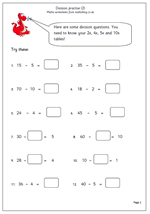being able to divide mentally is essential and is made much easier by knowing times tables.

Here is a follow up page to one published earlier, giving more practice with simple division. If children have a good knowledge of the 2x, 4x, 5x and 10x tables they should find these quite straightforward. The only potentially tricky ones are where the missing number is in the middle of the number sentence

eg 24 divided by ? = 6

This requires a good understanding of what the number sentence means, but all that is required to answer is a knowledge of ‘what multiplied by 6 makes 24’.

This worksheet can be found in our Year 3 Knowing Number Facts section.

Y3 division practice 2

## Pairs of numbers that make 20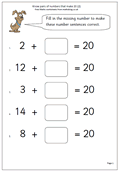Here we have a straightforward maths worksheet on knowing pairs of numbers that add up to twenty. This is suitable for year 1/2 children or those who are already very confident with knowing pairs of numbers that make ten.

If these facts are not known there are several ways of working the answers out, including:

1. Counting on from the smaller number.

2. Counting on from the smaller number up to 10 and then adding another 10. (If smaller number is below 10.)

3. Counting back from 20, which is trickier.

Know pairs that make 20 (pg 2)

## Resource of the week: year 1 investigate additionWelcome to a new term and plenty of new maths material coming up over the next few months.

From our current resources here is a nice little investigation for young children which will show how well they can organise their thinking and work in a logical way.

The question is simple: how many different ways can you score 8 when throwing two dice?

It’s always a good idea to sit down with your children when doing this type of activity. In this way you can ask questions which will help them clarify their thoughts. The sheet is designed to be used as a record of results. At first they might just keep rolling the dice and adding up the totals until a total of 8 is achieved. Other children might dive straight in with some answers eg 4 + 4 makes 8.

A good question to ask at the end is “How do you know that you have got all the possibilities?

Free Y1 maths worksheet: Investigate dice

## Revising times tables in Year 4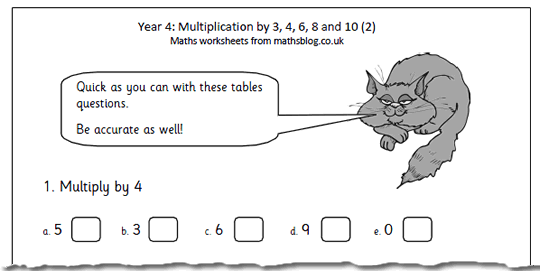What have we got today? It’s the second in our multiplying by 3, 4, 6, 8 and 10 worksheets, suitable for year 4 children or those who are beginning to know their tables.

The best way to learn tables is to recite them out loud eg ‘4 times 3 is 12, then shorten it to,  ‘4 3s are 12’, with the ultimate aim of being able to say the product for any two single digits without having to work it out.

During the process of learning times tables plenty of practice is needed and how well they have been learned can be seen by how quickly the page is correctly answered.

Multiplication 3x 4x 6x 8x 10_(pg 2)

## Year 2: Time. What can you do in one minute?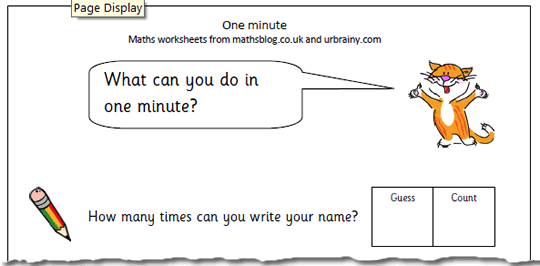Getting a clear concept of amount of time is important for young children. This page challenges children to complete a number of tasks in one minute, such as how tall a tower can be built using blocks/lego etc.

An important part of this is to make sure that they make an estimate/guess before starting which shows how realistic they are about time. One minute is a short time, but it is surprising how much can be achived in just 60 seconds!

This resource can be found in our Year 2 measurement section.

One minute

## Single step word problems for year 6Below are two pages of maths problems written in words. They are known as ‘single step operations’ as only one mathematical process is necessary to solve them. Children find word problems very difficult, but the one step type are much, much easier than the two step.Children need to be able to read and understand problems written in prose that include elements of real life, either at home or at school. They need to be able to see what processes are necessary to solve it and then lay out their answer clearly, giving some explanation. If they have had plenty of practice at writing their own number stories in earlier years they will now find these much easier.

Single step operations (pg 1)

Single step operations (pg 2)

## Year 5 Knowing multiplication facts to help with division.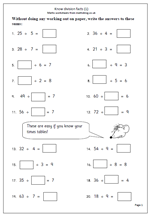There is no doubt that most children find division harder than multiplication, yet there is little real reason for this to be so. Most division questions can be turned on their head and knowledge of times tables used to work them out. For example:

30 ÷ 5 =??  can be thought as what number times 5 makes 30?

?? ÷ 6 = 7 can be thought of as 6 times 7.

60 ÷ ?? = 10 can be thought of as what number times 10 makes 60?

The key to success with division is to have a really good knowledge of times tables.

This worksheet looks at these types of division question, all with easy numbers and no remainders and is a good assessment sheet to see if division is understood and tables known. if times tables are not known sufficiently well children will spend more time than necessary trying to work these out.

This can be found in our Year 5 Knowing Number Facts category.

Know division facts (1)